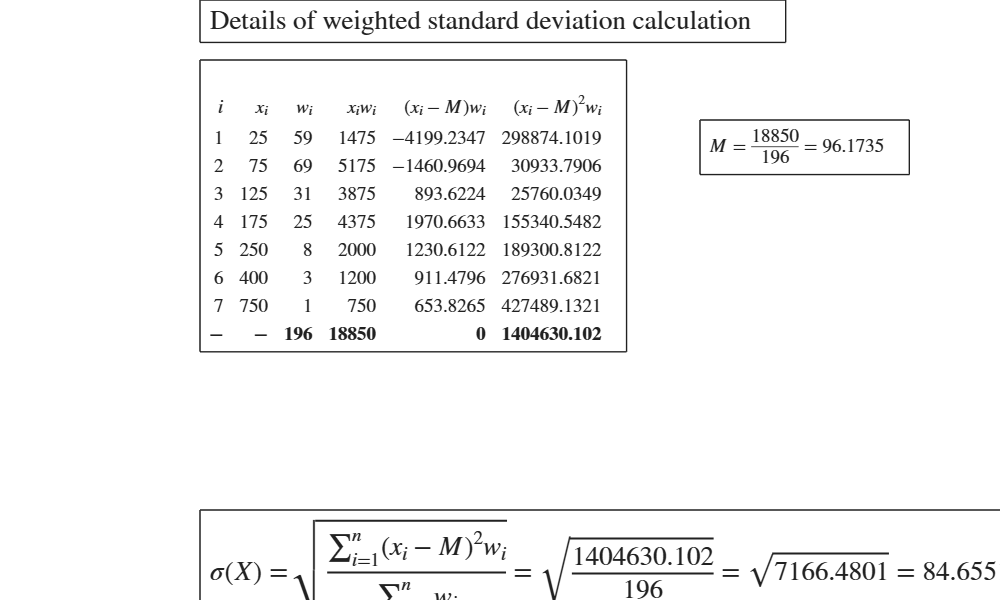# GUIstd

GUIstd shows the necessary calculations to obtain the standard deviation in a GUI.

## Syntax

• out=GUIstd(x)example
• out=GUIstd(x,w)example

## Description

 out =GUIstd(x) Standard deviation of the first 6 natural numbers.

 out =GUIstd(x, w) Calculation of standard deviation (using n as denominator).

## Examples

expand all

### Standard deviation of the first 6 natural numbers.

x=1:6;
GUIstd(x)

### Calculation of standard deviation (using n as denominator).

See page 9 of [CMR]

x=[427 492 445 444 476 470];
GUIstd(x,1)

## Related Examples

expand all

###Example of weighted standard deviation.

(See page 128 of [MRZ])

x=[25:50:175 250 400 750];
w=[59 69 31 25 8 3 1];
y=GUIstd(x,w);## Input Arguments

### x — vector of numeric data. Vector.

Vector containing strictly numerical data.

Data Types: double

### w — weights. Vector or scalar.

Vector of the same length of x containing the weights (frequencies) assigned to each observation or scalar which specifies the normalization to use.

If w=1 the denominator of the index corresponds to the number of observations.

If w=0 (default), the denominator of the index corresponds to the number of observations minus 1, If w is not supplied we assume that all observations have weight equal to 1 and the index is normalized with length(x)-1.

Example: 1:10 

Data Types: double

## Output Arguments

### out —detailed output to compute the index. Table

Table with n+1 rows (where n is the length of x) containing what is shown in the GUI. Last row contains the totals.

Milioli, M.A., Riani, M., Zani, S. (2019), "Introduzione all'analisi dei dati statistici (Quarta edizione ampliata)". [MRZ]

Cerioli, A., Milioli, M.A., Riani, M. (2016), "Esercizi di statistica (Quinta edizione)". [CMR]